Download PDF文章快速检索 高级检索

Four-rotor route planning based on the ant colony algorithm
MO Hongwei, MA Jingwen
College of Automation, Harbin Engineering University, Harbin 150001, China
Abstract: Given a four-rotor unmanned aerial vehicle's characteristics and complex flight environment, as well as the accuracy of the global positioning system in the environment model, the establishment of a 3D environment model based on elevation maps has reduced the probability of encountering obstacles. In terms of planning algorithms, most of the existing path planning algorithms can only plan 2D paths. Numerous 3D planning algorithms have complex computations and require much storage space. A global path is also difficult to plan. The advantages of the ant colony algorithm include distributed computing and swarm intelligence. Moreover, this algorithm has great potential in path planning. However, when the fundamental ant colony algorithm is used in a 3D track search, the two directly connected planes easily track straight through obstacles. The track then includes more nodes, and the fitness value becomes too large. The algorithm was improved to address these issues by proposing the strategy of converting the main direction to search and the simplified track strategy. Ant simulation results showed that the improved algorithm could avoid obstacles, reduce path length, and improve search efficiency.
Key words: four-rotor unmanned aerial vehicle     route planning     three-dimensional environment model     ant colony algorithm     strategy of converting the main direction to search     track simplification strategy

UAV三维航迹规划区域广，搜素空间巨大，所以要想找到一条最优的飞行轨迹就必定需要占用较长的收敛时间，同时还需要较大的存储空间，这对大部分无人机实际应用来说是不怎么实际的做法。近些年来，国外一些专家对航路规划算法归结出了三类主要的方法：传统算法，如栅格法、Voronoi图法；智能优化算法，如遗传算法、蚁群算法；其他算法，如动态规划算法。传统算法对障碍物要求比较理想化，对实际地形规划出的结果影响很大。动态规划算法，在局部路径上可以达到最优及适用于动态地图，但不能确保全局路径上也可以实现。 智能优化算法[1, 2, 3, 4]，如遗传算法，是一种依据生物界的进化规律演化而来的一种方法，它最重要的一个特点是不受函数求导的限制，在隐并行性方面和全局搜索方面有很大优势，在稳定性方面也有显著提高，但算法效率慢，不能适用于动态、真实的地图。

1 基于栅格图法的环境模型构建

 $$N = {{{X_{\max }} - {X_{\min }}} \over {{X_{Gid}}}} \times {{{Y_{\max }} - {Y_{\min }}} \over {{Y_{Gid}}}}$$ (1)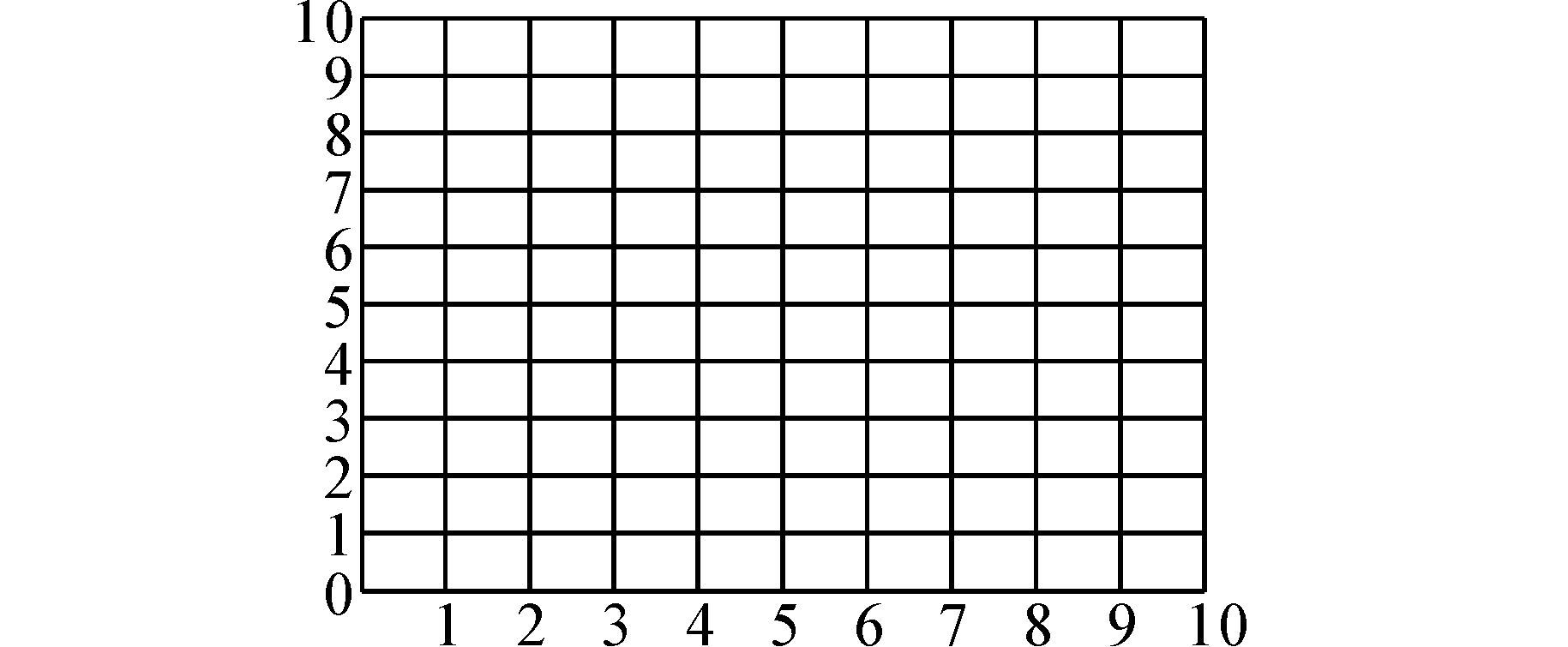图 1 二维栅格图Fig. 1 Two-dimensional raster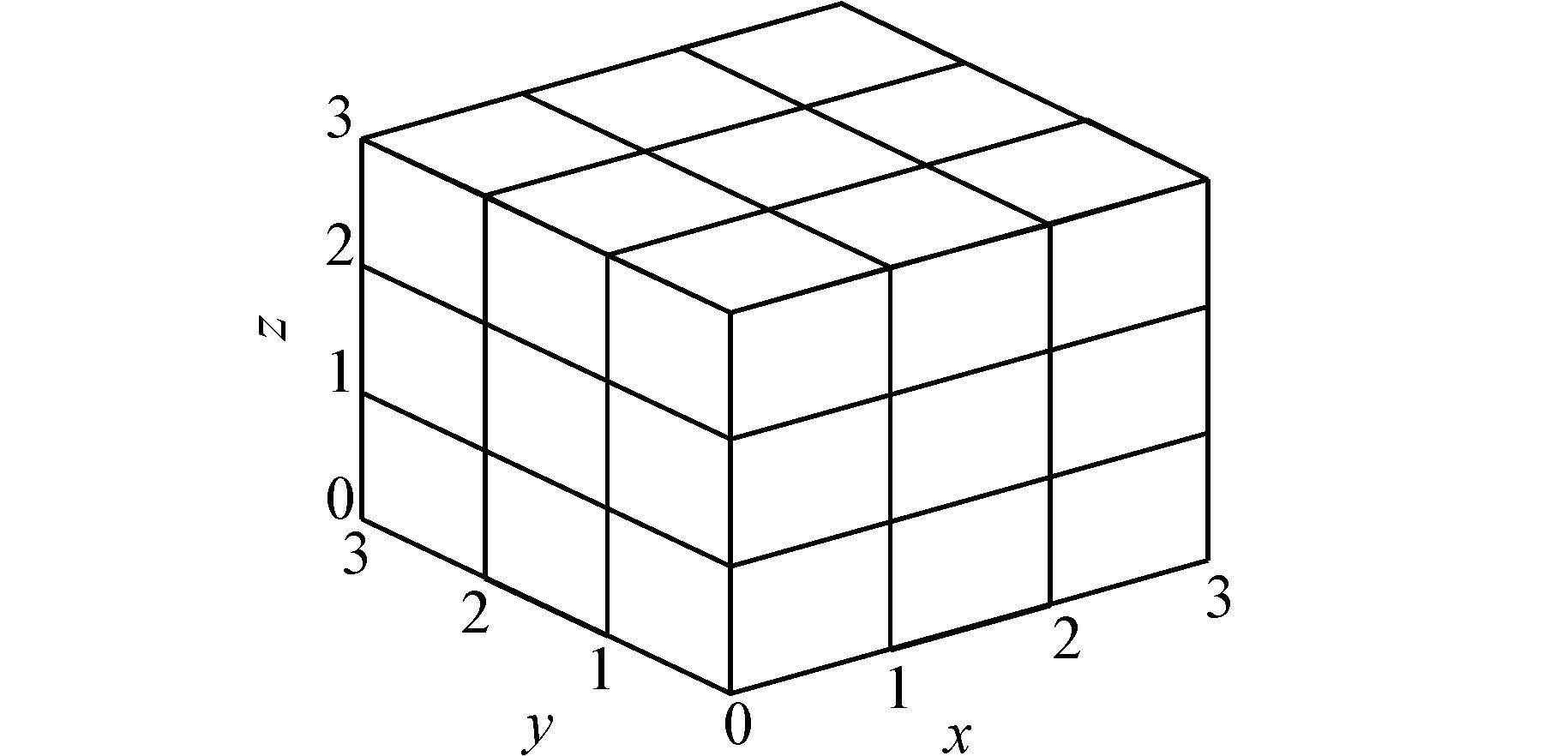图 2 三维栅格图Fig. 2 Three-dimensional raster

 $$N = {{{X_{\max }} - {X_{\min }}} \over {{X_{Gid}}}} \times {{{Y_{\max }} - {Y_{\min }}} \over {{Y_{Gid}}}} \times {{{Z_{\max }} - {Z_{\min }}} \over {{Z_{Gid}}}}$$ (2)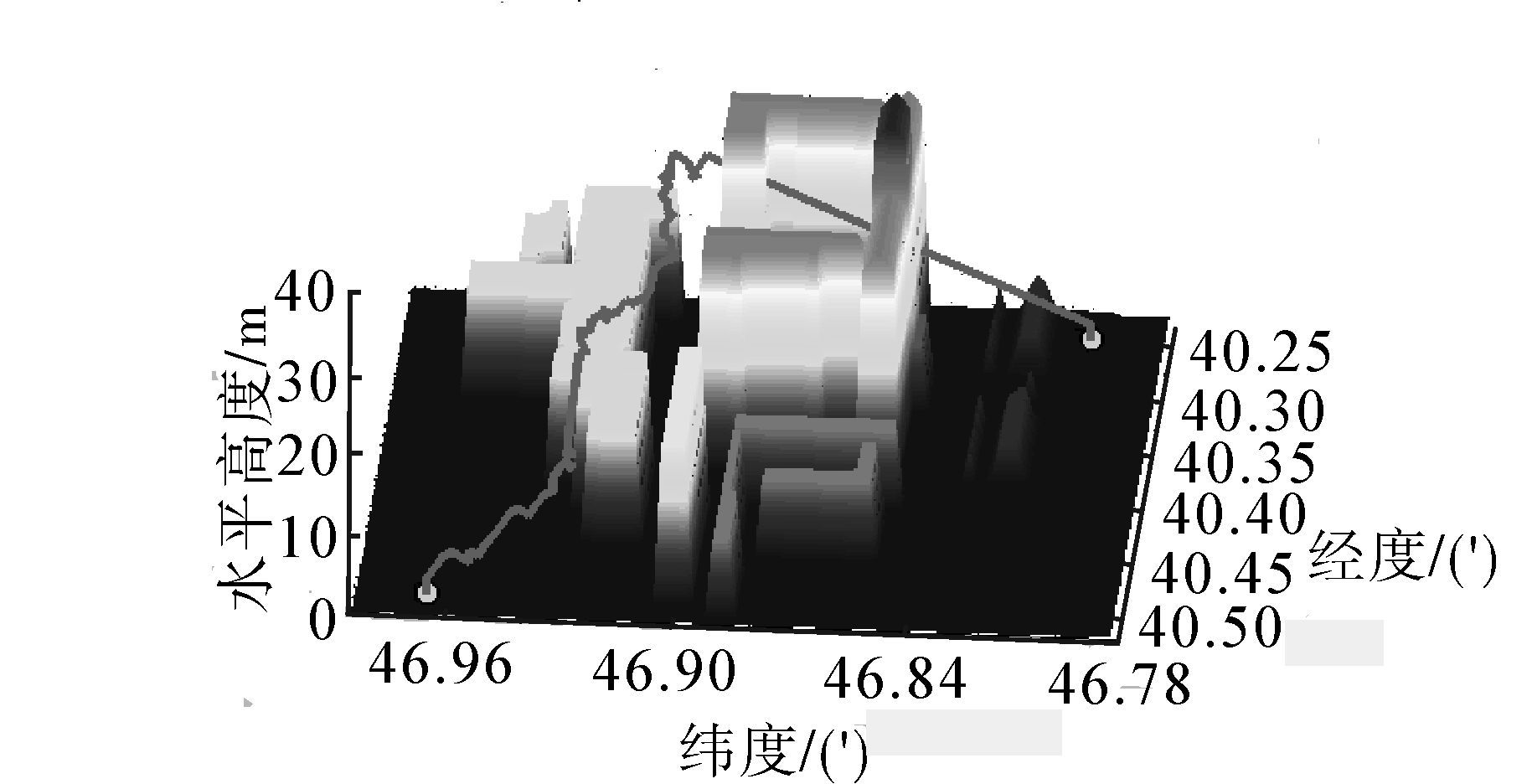图 3 三维图Fig. 3 Three-dimensional map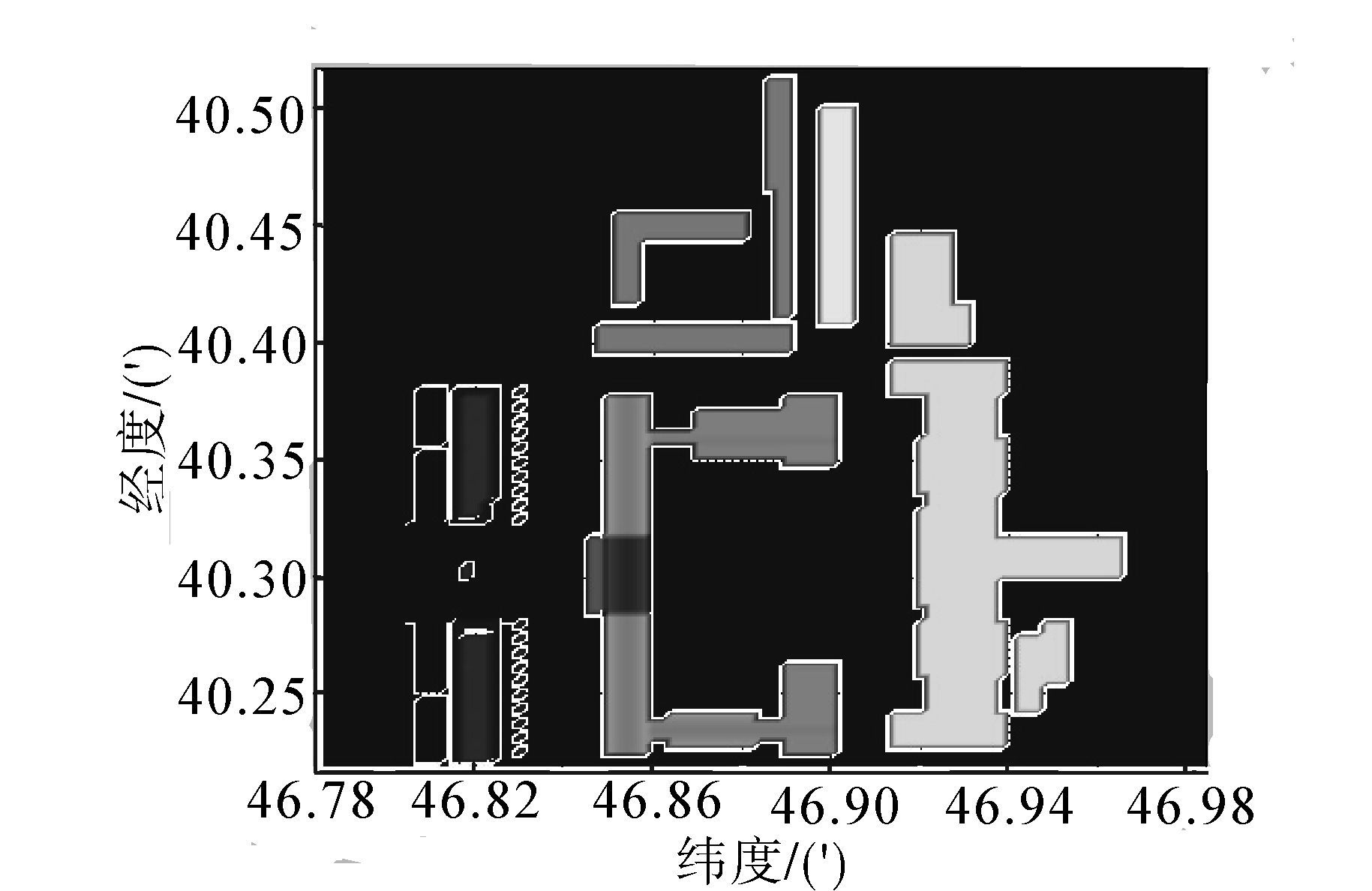图 4 投影图Fig. 4 Projection map
2 三维蚁群算法

2.1 算法的数学模型

 $$P(X,Y,Z) = \left\{ \matrix{ {{\tau (X,Y,Z) * H(X,Y,Z)} \over {\sum\limits_{}^{} {\tau (X,Y,Z) * H(X,Y,Z)} }} \hfill \cr (X,Y,Z) \in Allowe{d_i} \hfill \cr 0,(X,Y,Z) \in Allowe{d_i} \hfill \cr} \right.$$ (3)

 \eqalign{ & H(X,Y,Z) = \cr & D{(X,Y,Z)^{\omega 1}} \times S{(X,Y,Z)^{\omega 2}} \times Q{(X,Y,Z)^{\omega 3}} \cr} (4)

 $$D(X,Y,Z) = \sqrt {{{({X_i} - X)}^2} + {{({Y_i} - Y)}^2} + {{({Z_i} - Z)}^2}}$$ (5)
S(X,Y,Z)表示安全性因素，促使蚂蚁选择安全点。当前栅格(Xi,Yi,Zi)和(X,Y,Z)是不可连通的，或者栅格(X,Y,Z)内有障碍物，则称该栅格是不可达的。S(X,Y,Z)的计算公式为
 $$S(X,Y,Z) = \left\{ \matrix{ 1,(X,Y,Z)是可达点 \hfill \cr 0,其他 \hfill \cr} \right.$$ (6)

 \eqalign{ & Q(X,Y,Z) = \cr & \sqrt {{{({X_i} - {X_{end}})}^2} + {{({Y_i} - {Y_{end}})}^2} + {{({Z_i} - {Z_{end}})}^2}} \cr} (7)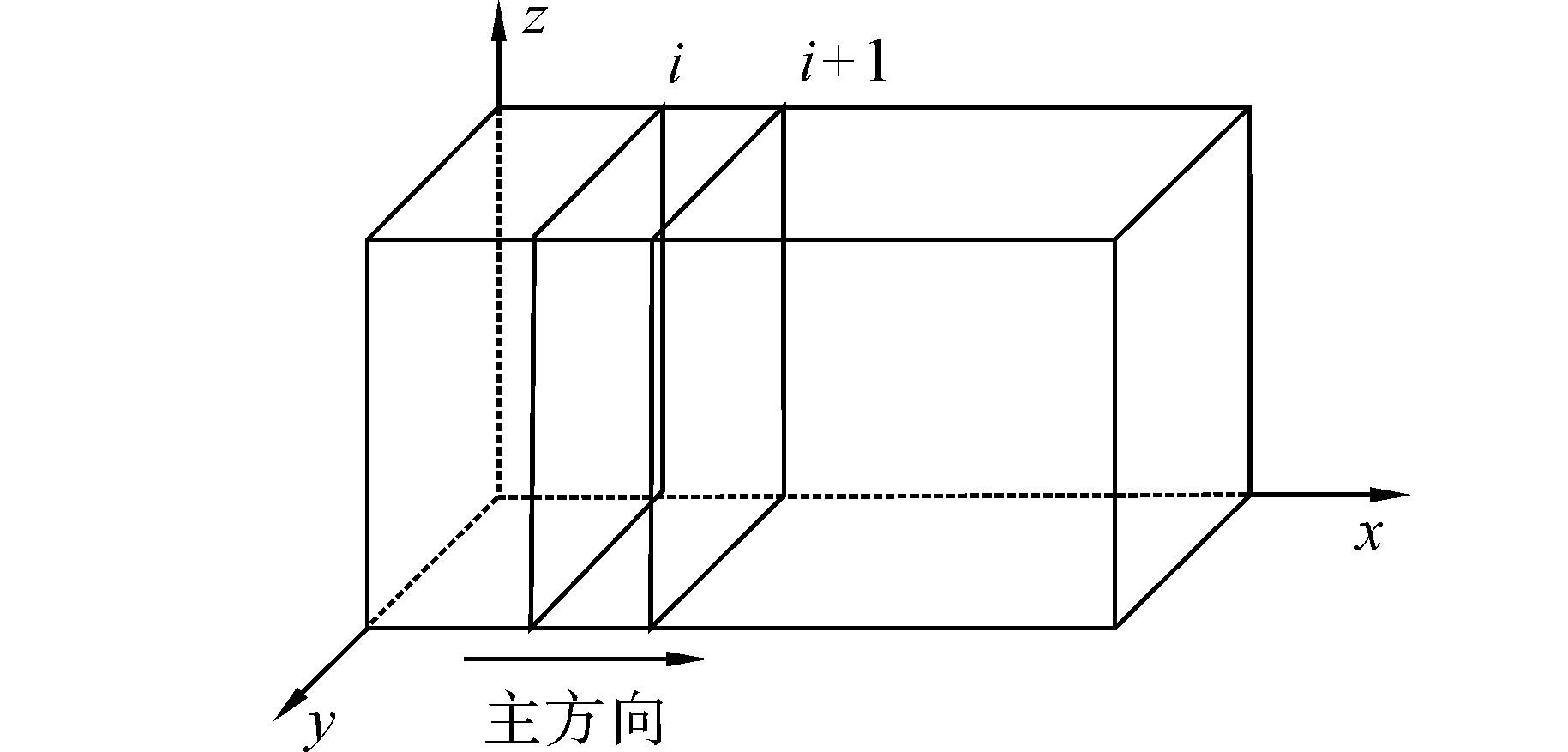图 5 主方向选取Fig. 5 Select the main direction

 $${\tau _{x,y,z}} = (1 - \zeta ){\tau _{x,y,z}}$$ (8)

 $${\tau _{x,y,z}} = (1 - p){\tau _{x,y,z}} + p\Delta {\tau _{x,y,z}}$$ (9)
 $$\Delta {\tau _{x,y,z}} = {K \over {\min (length(m))}}$$ (10)

2.2 算法的主要流程

1)参数初始化设置

①起始点的确定。当把四旋翼无人机放置在规划空间中的某一点时，放置四旋翼的位置不一定恰好就是栅格图中栅格的位置，这时就需要把该四旋翼无人机所在的栅格的栅格坐标作为航迹规划的起始点。那么，三维栅格地图原点坐标为

 $${X_{start}} = ceil({{{S_{lat}} - {X_{Gridstart}}} \over {{X_{Grid}}}}) \times {X_{Grid}} + {X_{Gridstart}}$$ (11)
 $${Y_{start}} = ceil({{{S_{lat}} - {Y_{Gridstart}}} \over {{Y_{Grid}}}}) \times {Y_{Grid}} + {Y_{Gridstart}}$$ (12)
 $${Z_{start}} = ceil({{{S_{lat}} - {Z_{Gridstart}}} \over {{Z_{Grid}}}}) \times {Z_{Grid}} + {Z_{Gridstart}}$$ (13)

②主方向选取。主方向的选取主要就是以纬度方向为主方向还是以经度方向为主方向的问题。选择两个方向之中栅格变化数最多的方向作为四旋翼无人机航迹规划主方向。主方向选定后还要知道主方向上从起始点到终止点坐标值变化趋势，即坐标值是增加还是减少。如果坐标值增加，那么当从当前平面到下一平面上主方向上坐标值加1个单位值；否则坐标值减1个单位值。假设A方向为主方向，那么从Πi到Πi+1坐标变化为

 $${A_i} + 1 = \left\{ \matrix{ {A_i} + {A_{Grid}},{A_{start}} \le {A_{end}} \hfill \cr {A_i} - {A_{Grid}},其他 \hfill \cr} \right.$$ (14)

③种群数选取。

④迭代次数的选择。

⑤航迹搜寻范围选取。如果主方向是A，非主方向的那个纬度或经度方向是B，那么从平面Πi到平面Πi+1，对于B方向上以Yi为中心从Yi-bcmaxYi+bcmax范围内的点都是可选择作为Yi+1点。同样，对于Z方向上来说以Zi为中心从Zi-hcmaxZi+hcmax范围内的点都是可选择作为Zi+1点。

⑥信息素初始化。在初始时刻，把三维栅格环境地图中的所有栅格信息素值设置为固定值。

2)航迹搜索

3)图形绘制

2.3 算法的改进 2.3.1 变主方向搜索策略

 $${{X - {X_1}} \over {{X_2} - {X_1}}} = {{Y - {Y_1}} \over {{Y_2} - {Y_1}}} = {{Z - {Z_1}} \over {{Z_2} - {Z_1}}}$$ (15)

 $$\left\{ \matrix{ Y = ({Y_2} - {Y_1}) \times (X - {X_1})/({X_2} - {X_1}) + {Y_1} \hfill \cr Z = ({Z_2} - {Z_1}) \times (Y - {Y_1})/({Y_2} - {Y_1}) + {Z_1} \hfill \cr} \right.$$ (16)

 $$\left\{ \matrix{ X = ({X_2} - {X_1}) \times (Y - {Y_1})/({Y_2} - {Y_1}) + {X_1} \hfill \cr Z = ({Z_2} - {Z_1}) \times (Y - {Y_1})/({Y_2} - {Y_1}) + {Z_1} \hfill \cr} \right.$$ (17)

a)X1=X2Y1Y2关系任意；

b)Y1=Y2X1X2关系任意；

c)X1>X2Y1>Y2

d)X1>X2Y1<Y2

e)X1<X2Y1>Y2

f)X1<X2Y1<Y2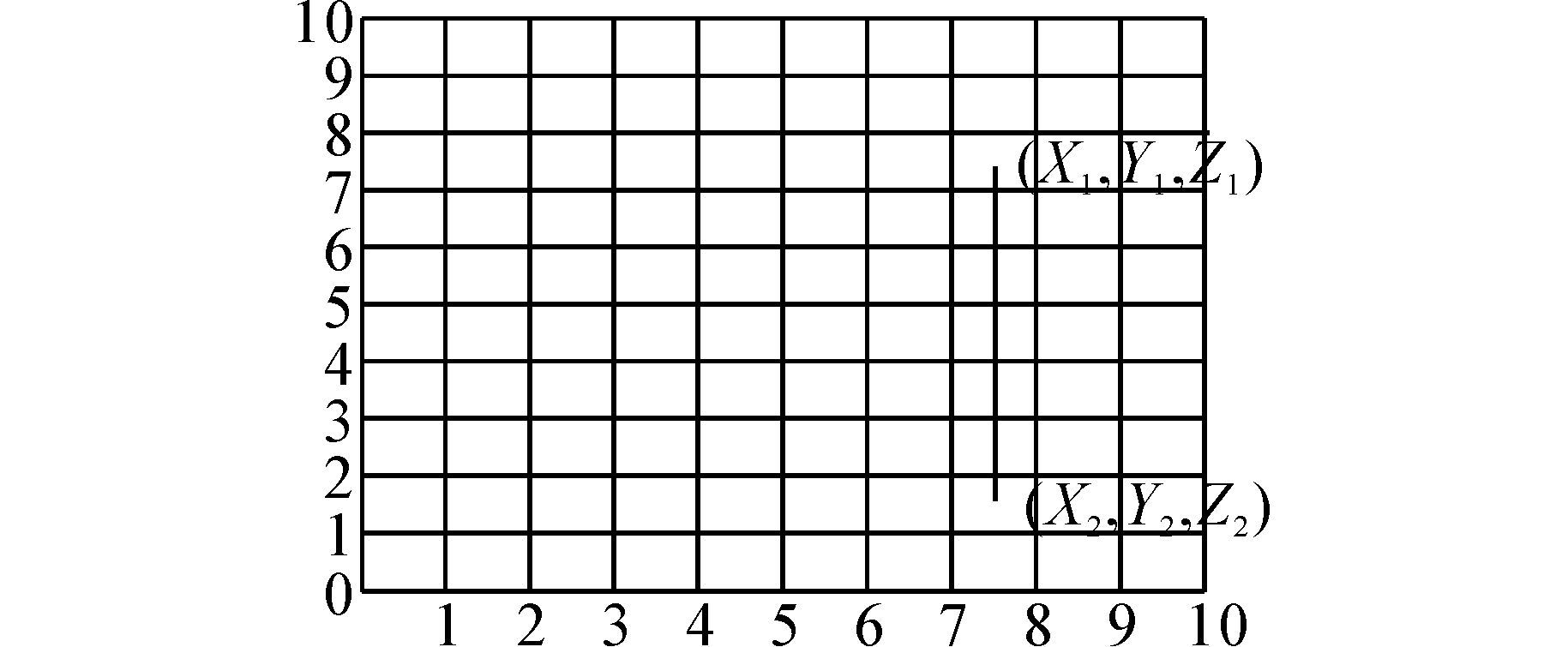图 6 X1=X2，Y1和Y2关系任意Fig. 6 X1=X2,Y1 and Y2 arbitrary relationship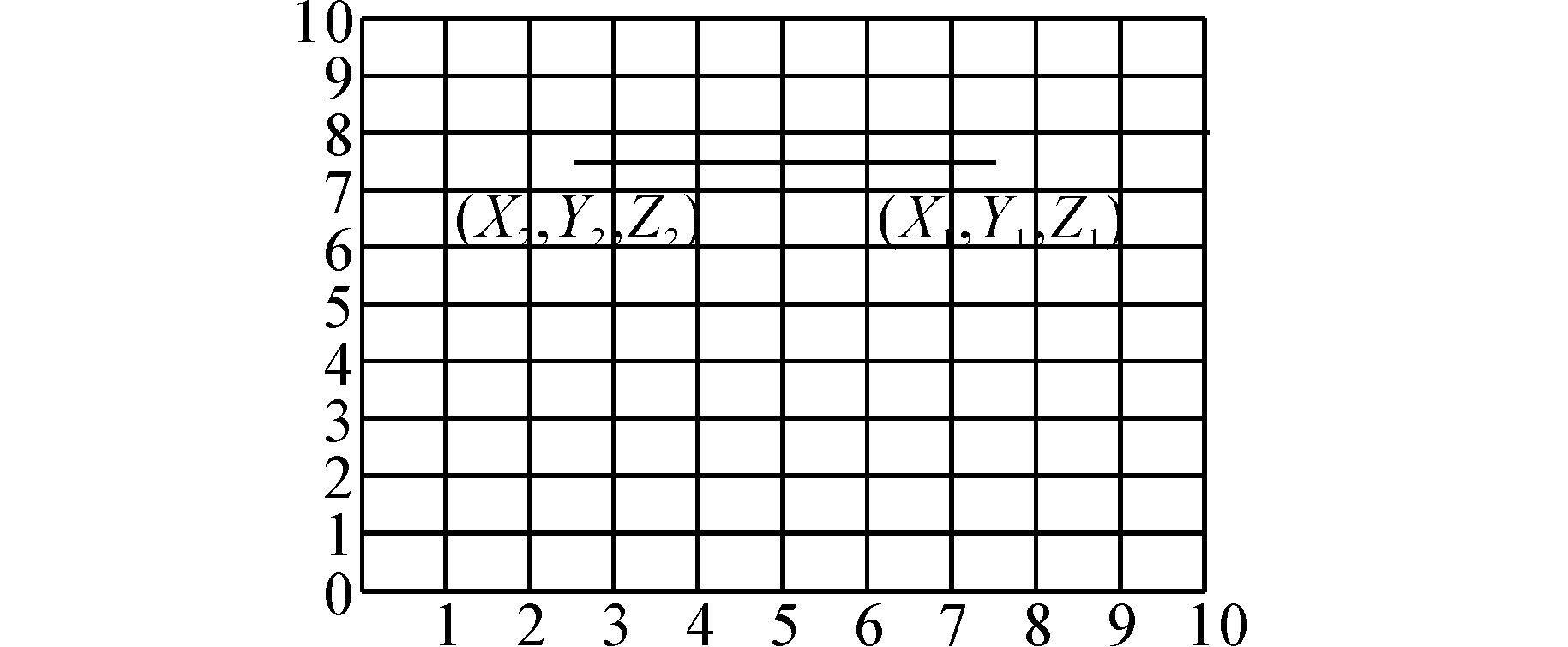图 7 Y1=Y2，X1和X2关系任意Fig. 7 Y1=Y2,X1 and X2 arbitrary relationship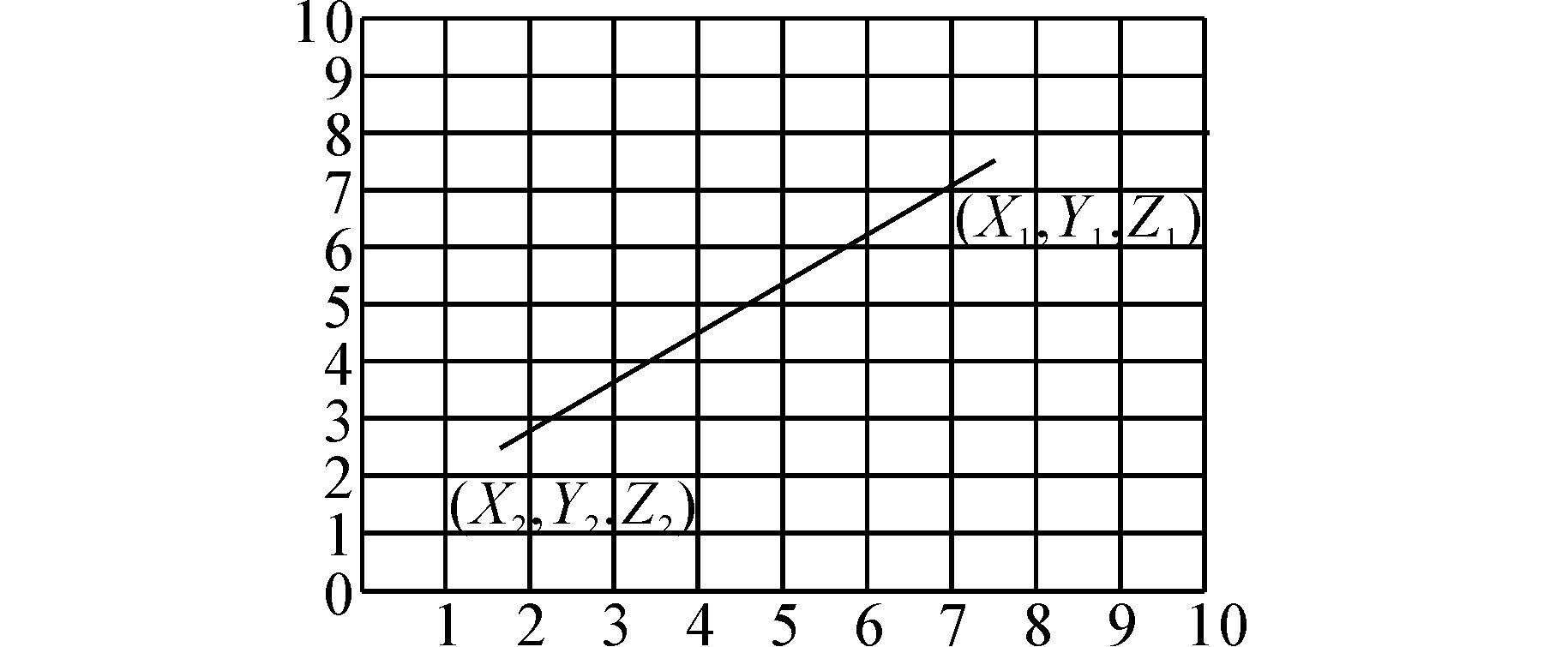图 8 X1>X2，Y1>Y2Fig. 8 X1>X2,Y1 >Y2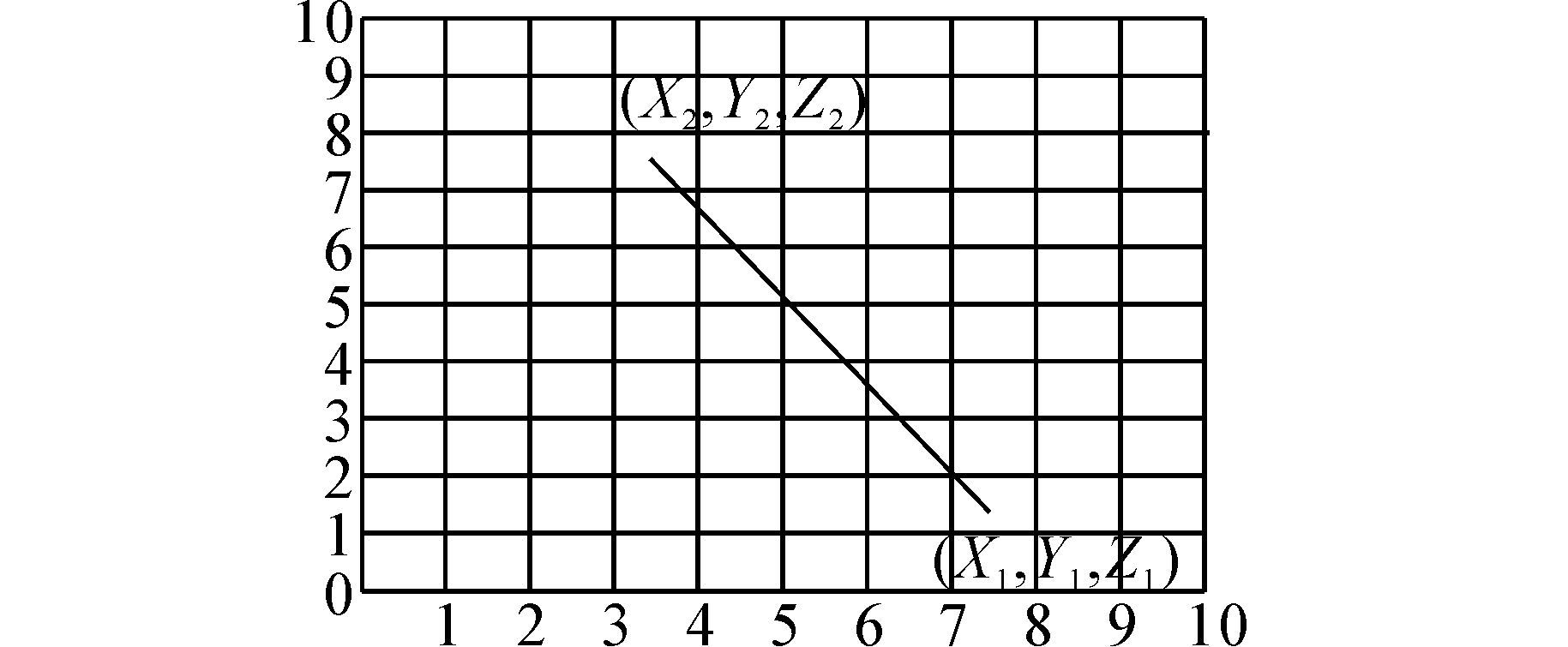图 9 X1>X2，Y1X2,Y1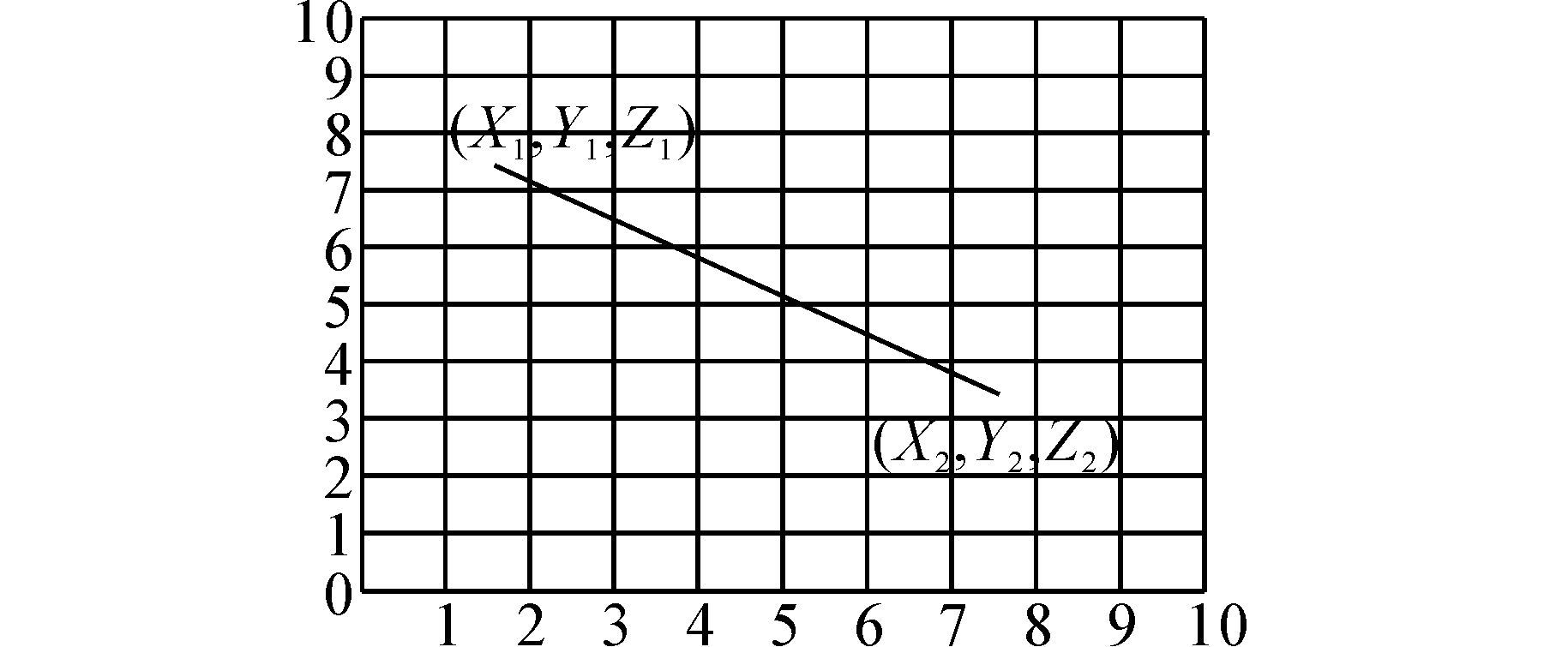图 10 X1Y2Fig. 10 X1Y2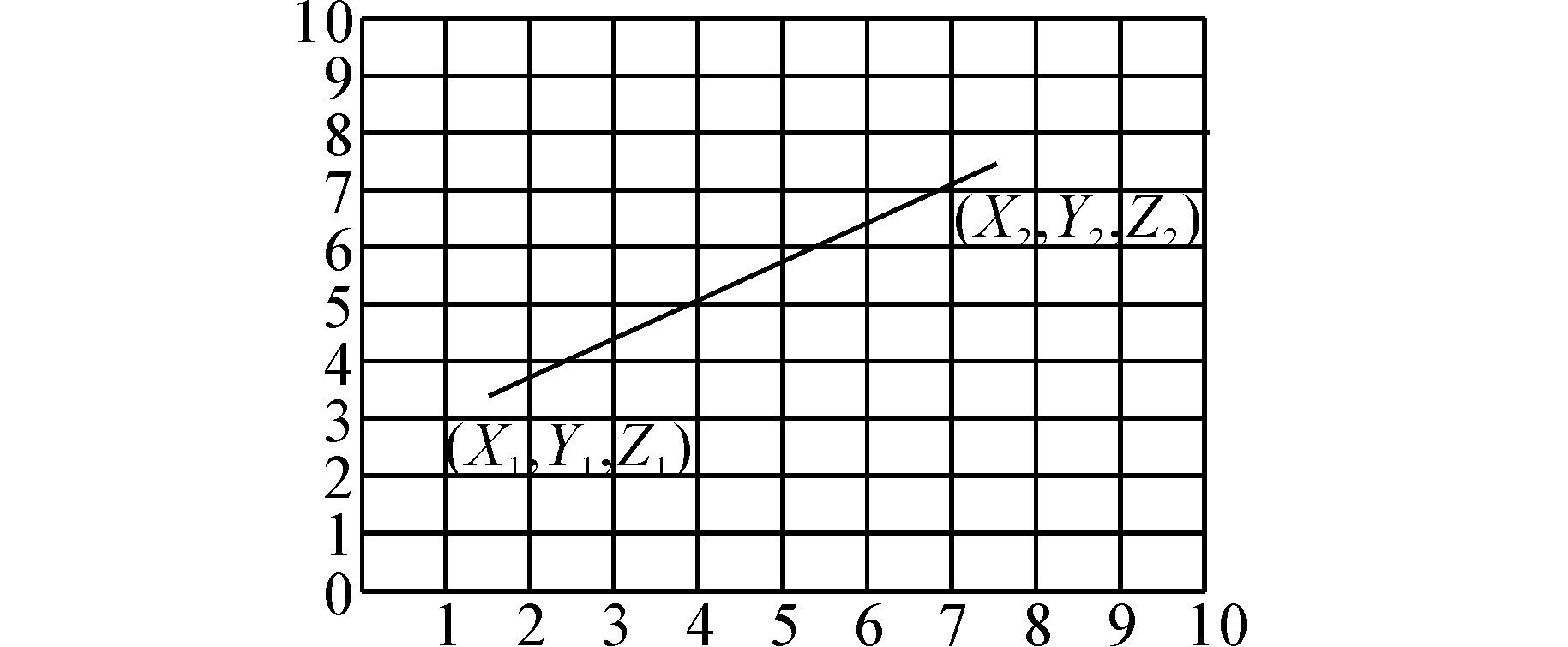图 11 X1

2.3.2 简化航迹策略

3 三维粒子群算法 3.1 粒子群算法的主要流程

1)确定起始点和终止点，对粒子群种群数、迭代次数等参数进行设定；

2)搜索num条可行航路，根据适应度函数计算个航路的适应度值，选取适应度值最小的航路R，根据主方向选取的不同将航路分成d段；

3)根据主方向的不同对速度进行分段初始化，由初始速度和航路R找出PopNum条航路作为各个粒子的初始航路；

4)根据粒子群初始航路计算各粒子的局部最优航路，再比较粒子群的局部最优航路找出适应度值最小的航路作为全局最优航路；

5)迭代开始，迭代次数k=0；

6)粒子群i=0；

7)根据迭代次数计算每一代ω的值，根据当前全局最优航路和局部最优航路对粒子群速度进行分段更新，更新完速度之后再对粒子群中各粒子的位置进行更新；

8)计算粒子i的新的航路的适应度值与粒子的局部最优值进行比较，如果当前航路的适应度值小于粒子i的最优航路的适应度值，以当前航路替换最优航路成为粒子i的新的最优航路；

9)如果i<PopNum，i值加1，重复7)，否则执行10)；

10)更新完所有粒子的位置后比较全局最优航路适应度值和粒子群当前局部最优航路适应度值，如果有某粒子的局部最优航路的适应度值小于全局最优航路的适应度值就用该粒子的航路代替全局最优航路成为新的全局最优航路；

11)如果k<Nk值加1，重复6)，否则转12)；

12)绘制三维地图和三维航迹。

4 仿真与分析 4.1 三维蚁群算法仿真分析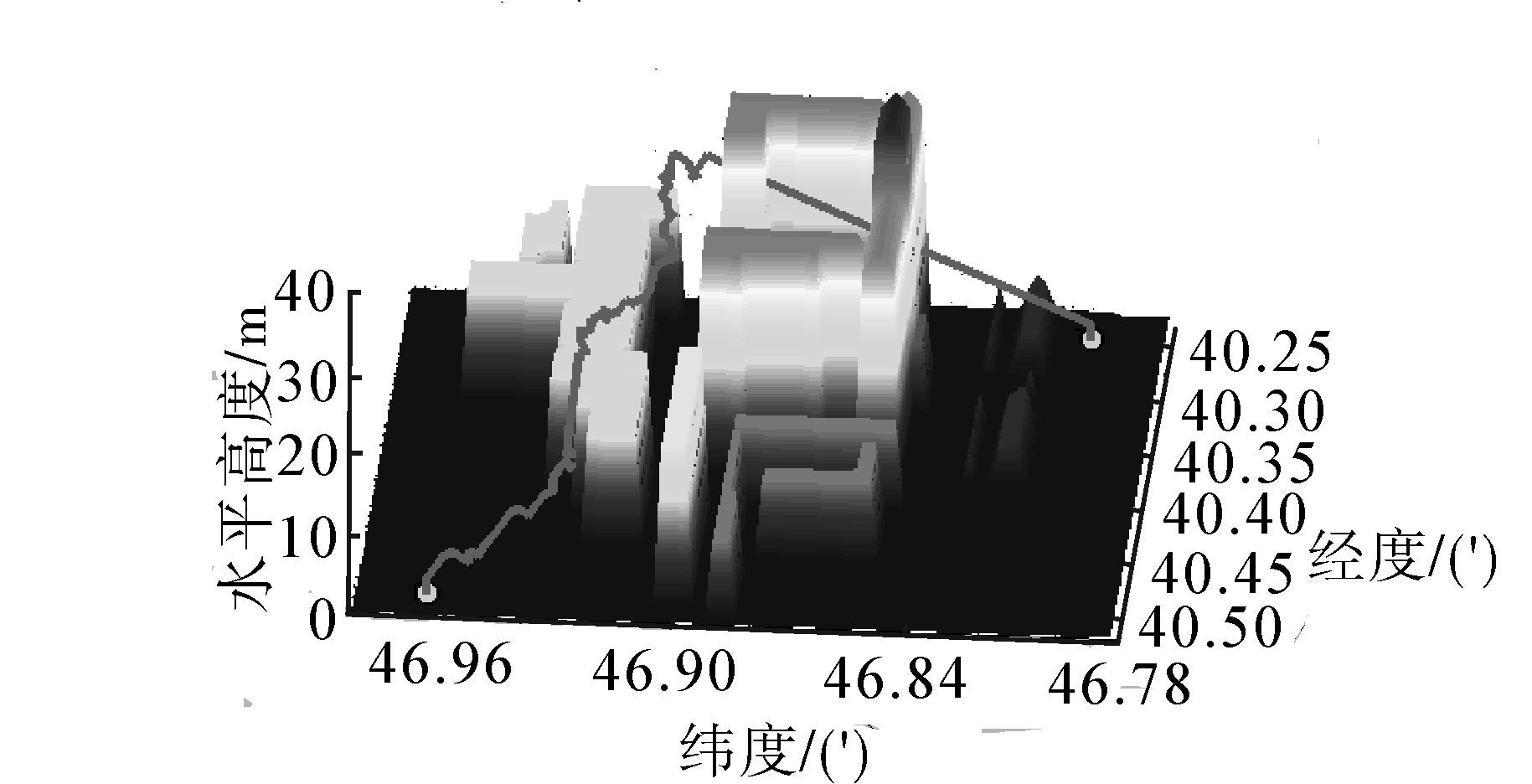图 12 蚁群算法搜索到的三维航迹图形Fig. 12 Three-dimensional graphics searched by ant colony algorithm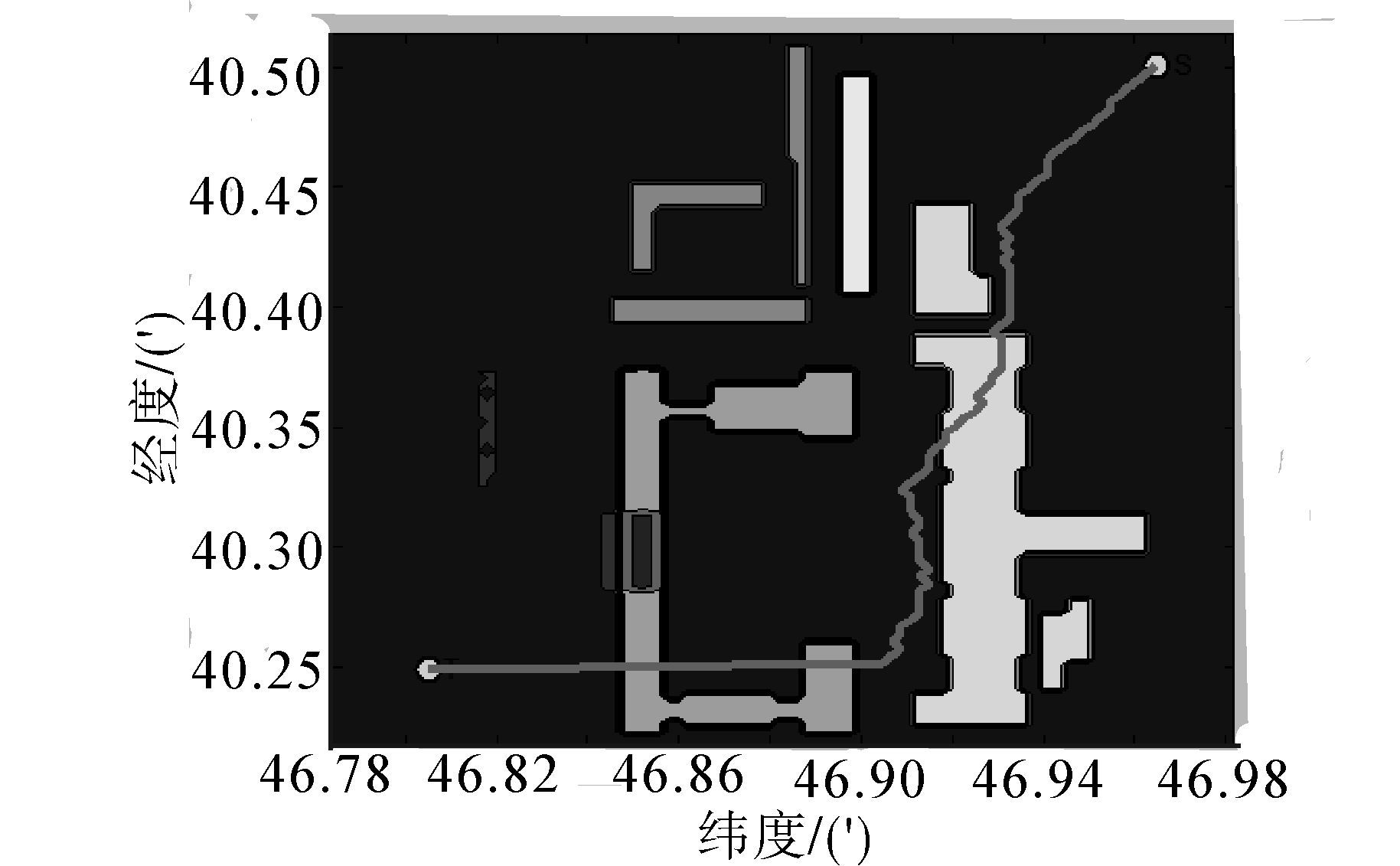图 13 三维航迹在二维平面上的投影Fig. 13 Three-dimensional trajectory projected onatwo-dimensional plane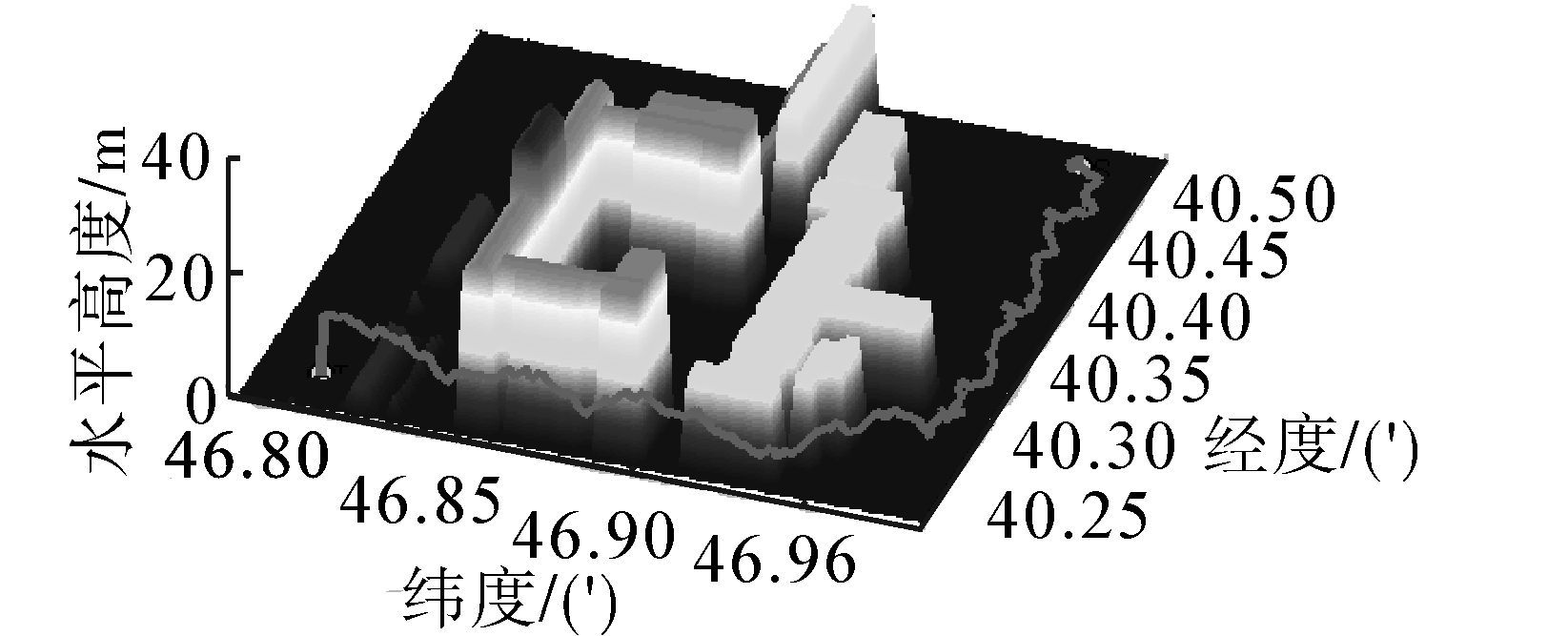图 14 加入变主方向策略后的三维航迹图Fig. 14 Three-dimensional graphics including the strategy of converting the main direction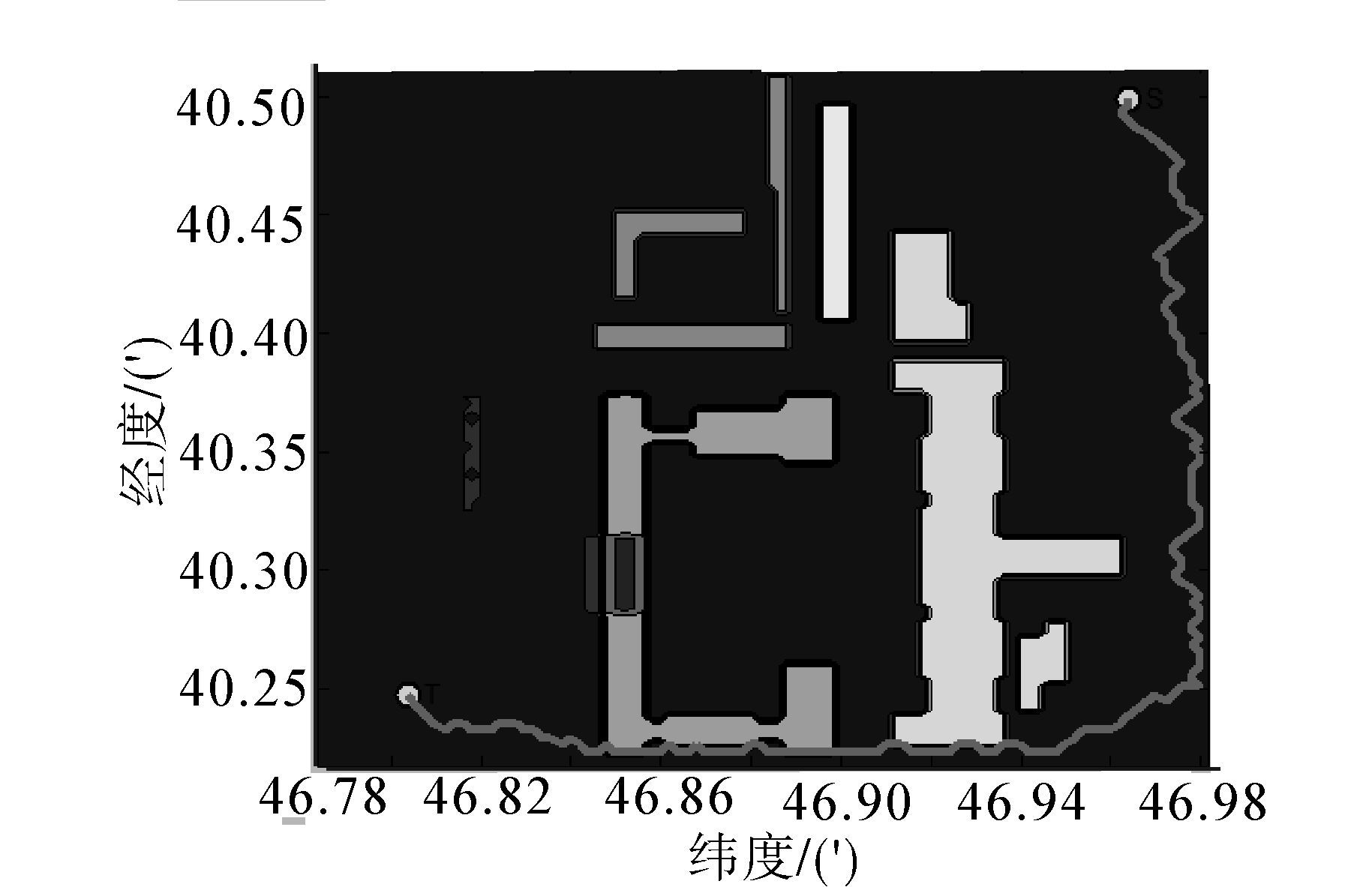图 15 加入变主方向策略的航迹二维投影图Fig. 15 The two-dimensional projection of track including the strategy of converting the main direction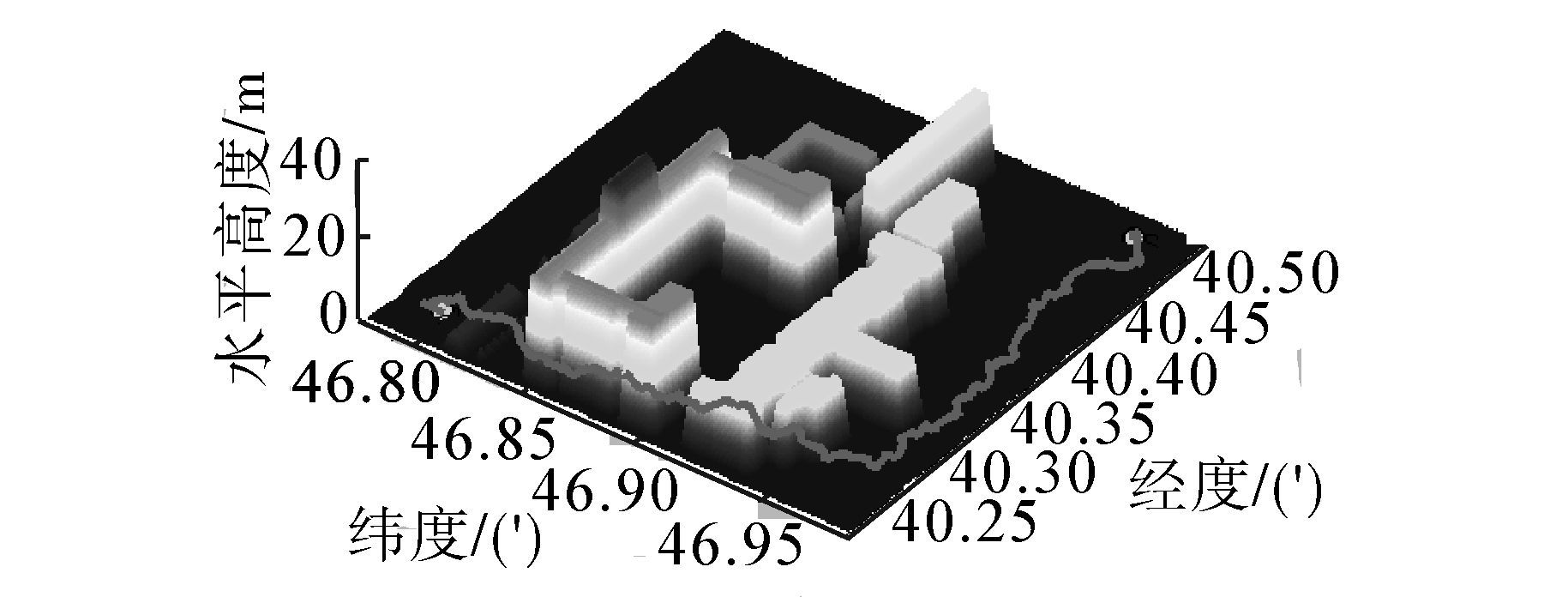图 16 未使用简化策略的三维航迹图Fig. 16 Three-dimensional graphics without simplification strategy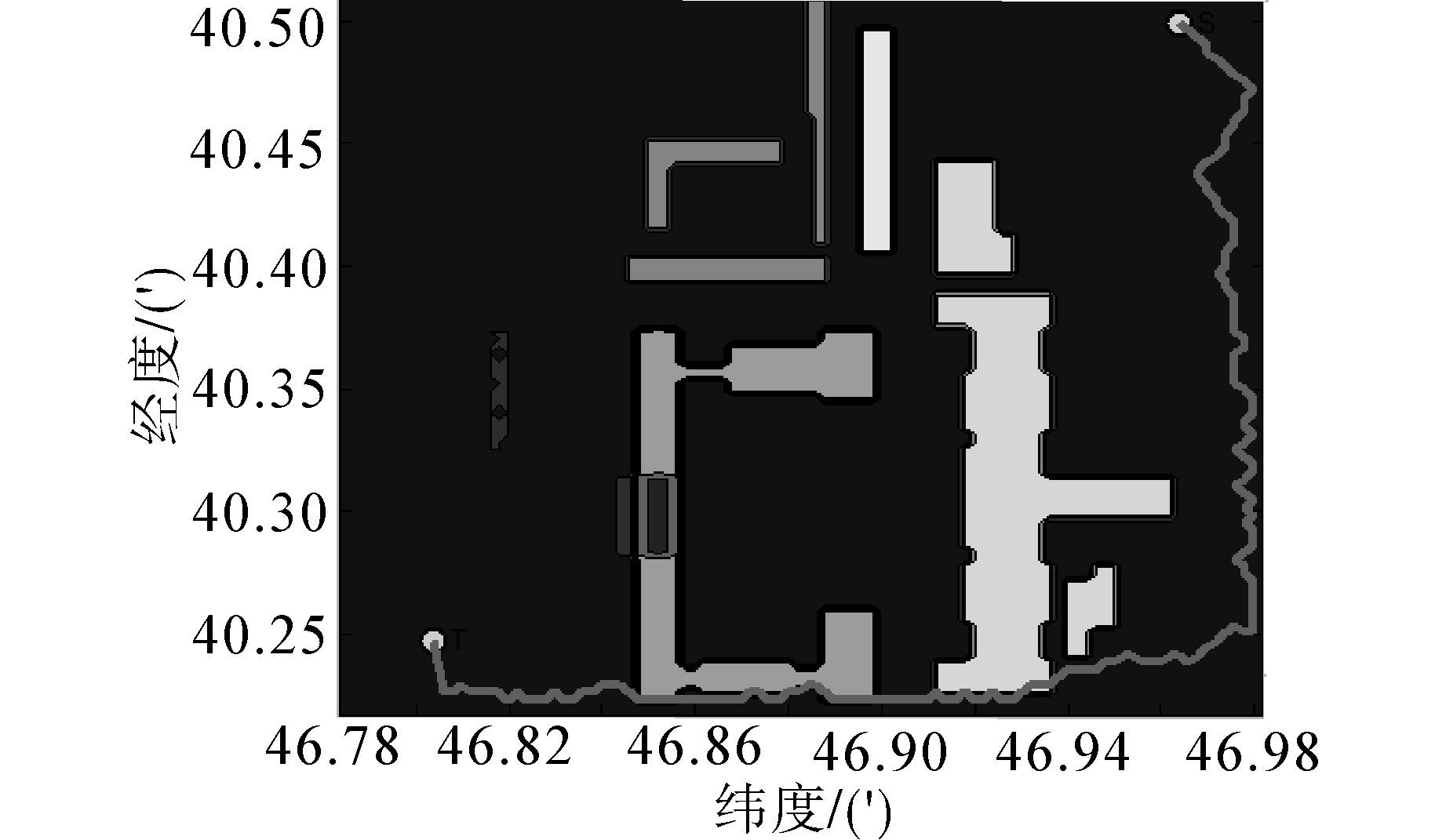图 17 未使用简化策略的航迹二维投影图Fig. 17 The two-dimensional projection of track without simplification strategy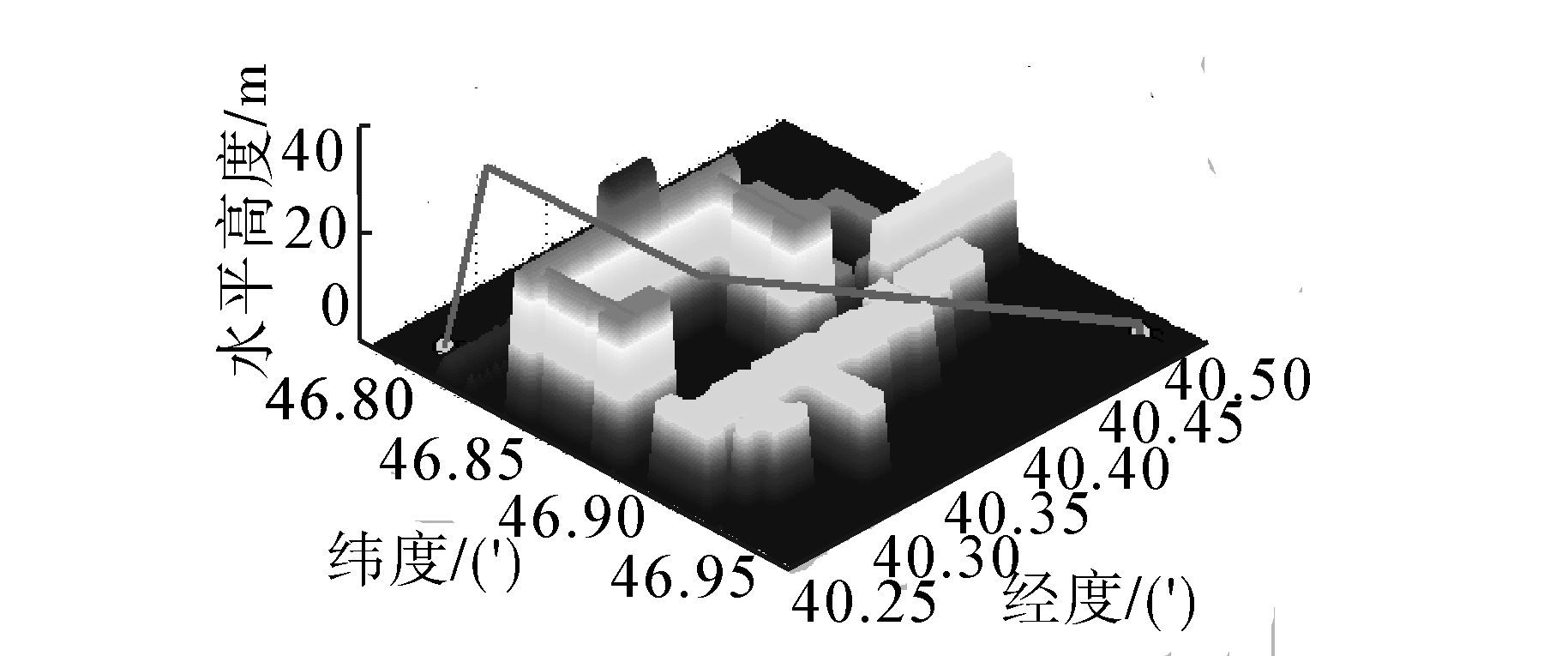图 18 使用简化策略的三维航迹图Fig. 18 Three-dimensional graphics with simplification strategy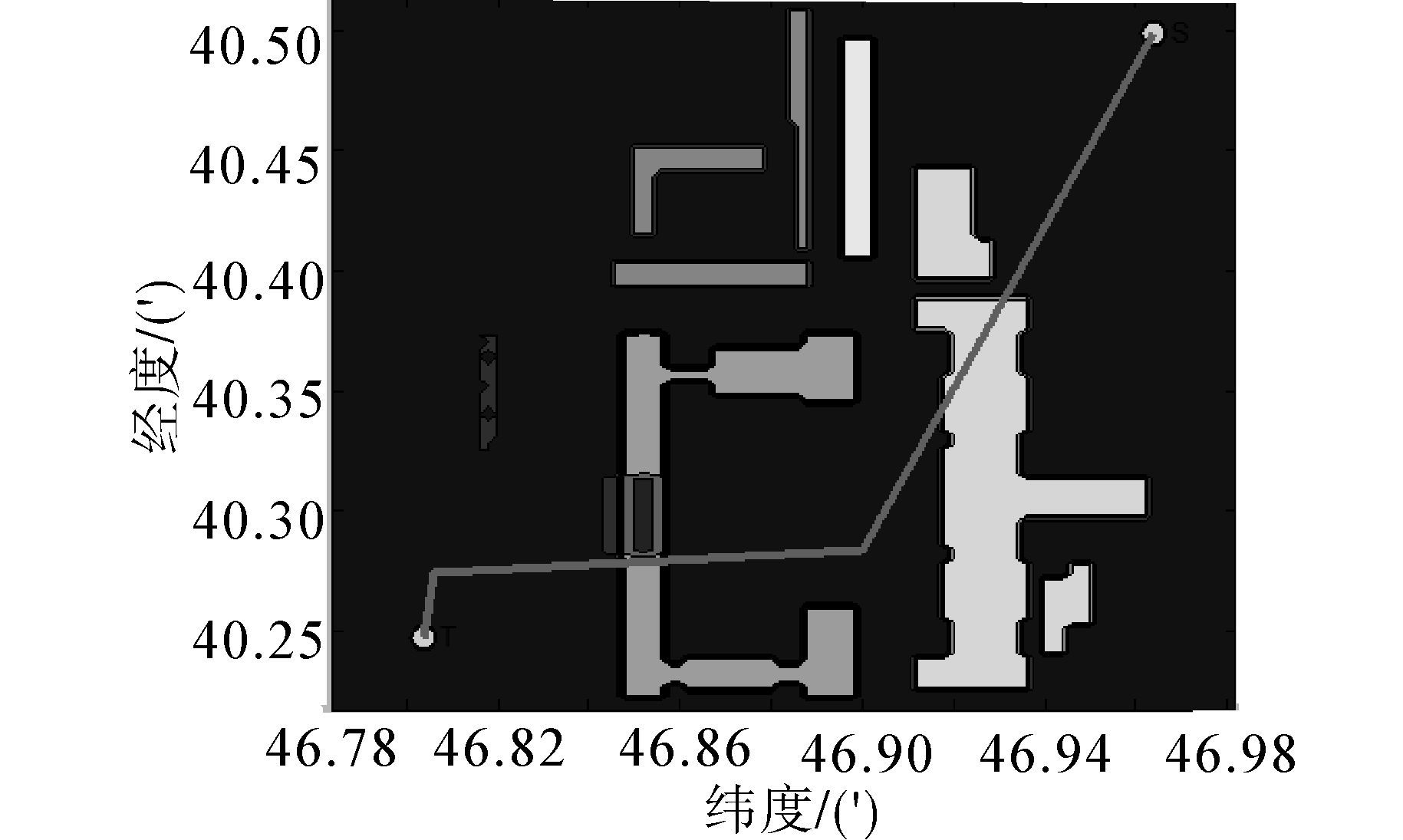图 19 使用简化策略的航迹二维投影图Fig. 19 The two-dimensional projection of track with simplification strategy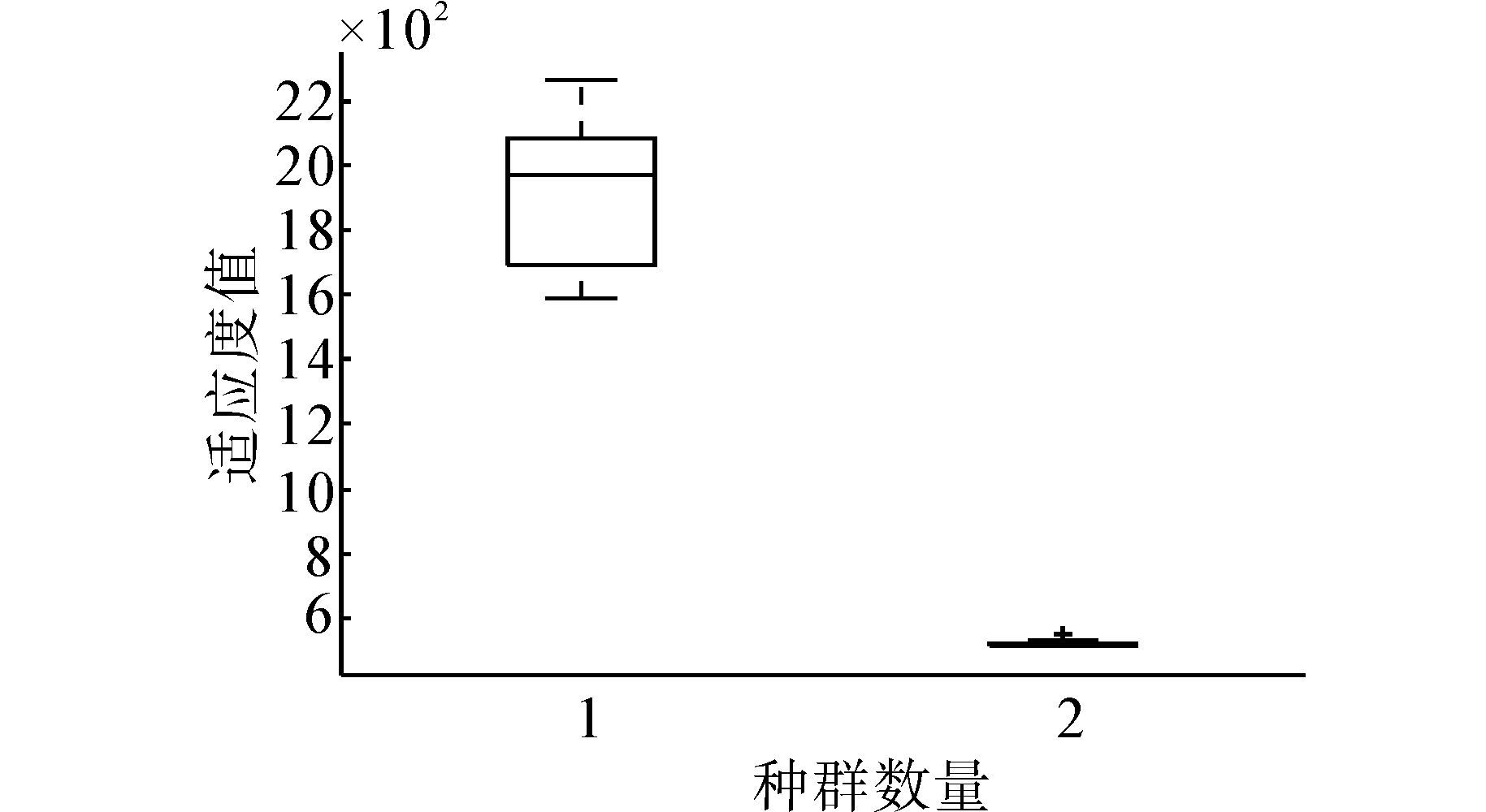图 20 简化前后适应度值统计Fig. 20 Statistics of fitness value with and without simplification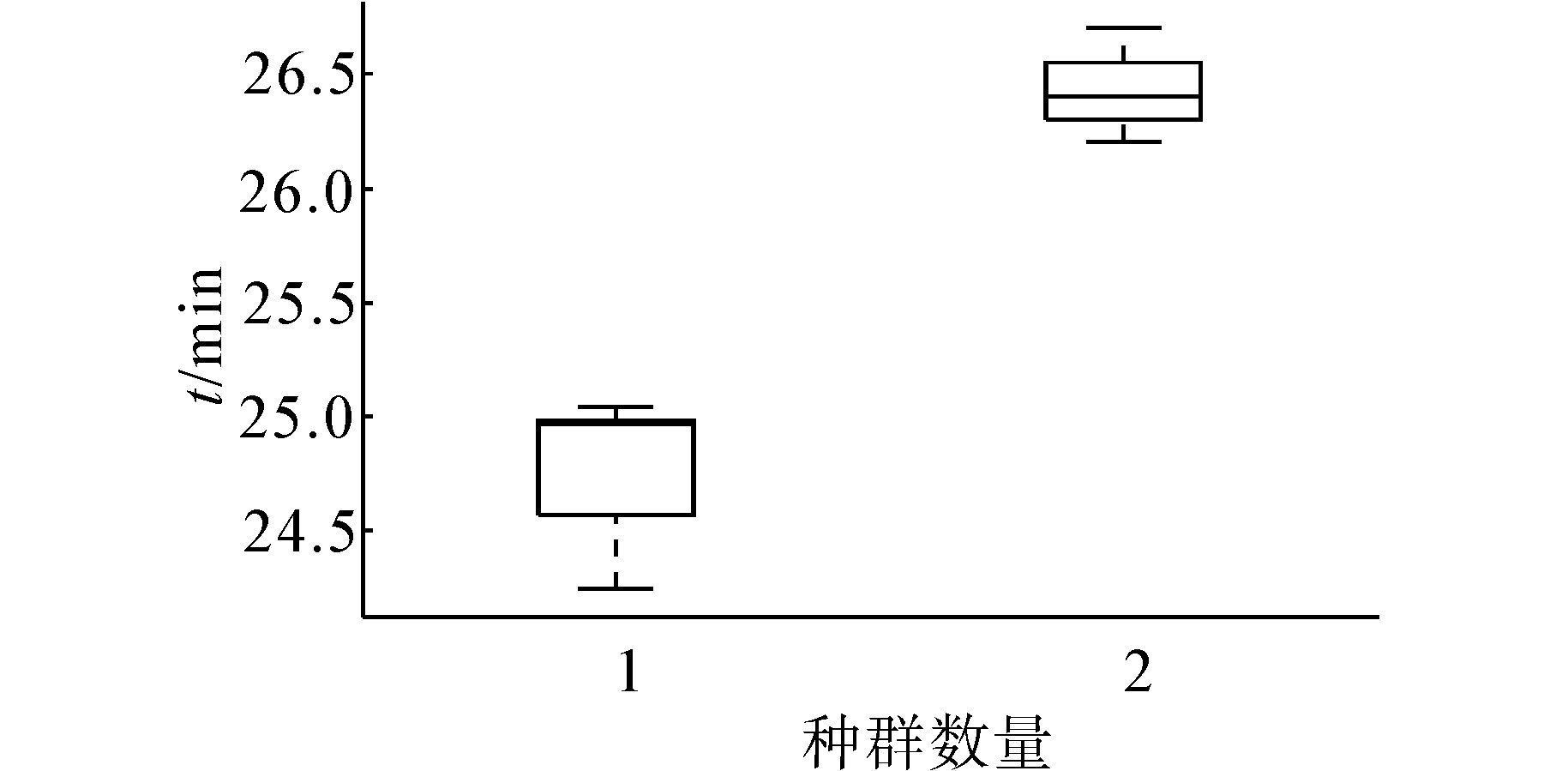图 21 简化前后搜索时间统计Fig. 21 Statistics of searching time with and without simplification

4.2 三维蚁群算法与三维粒子群算法对比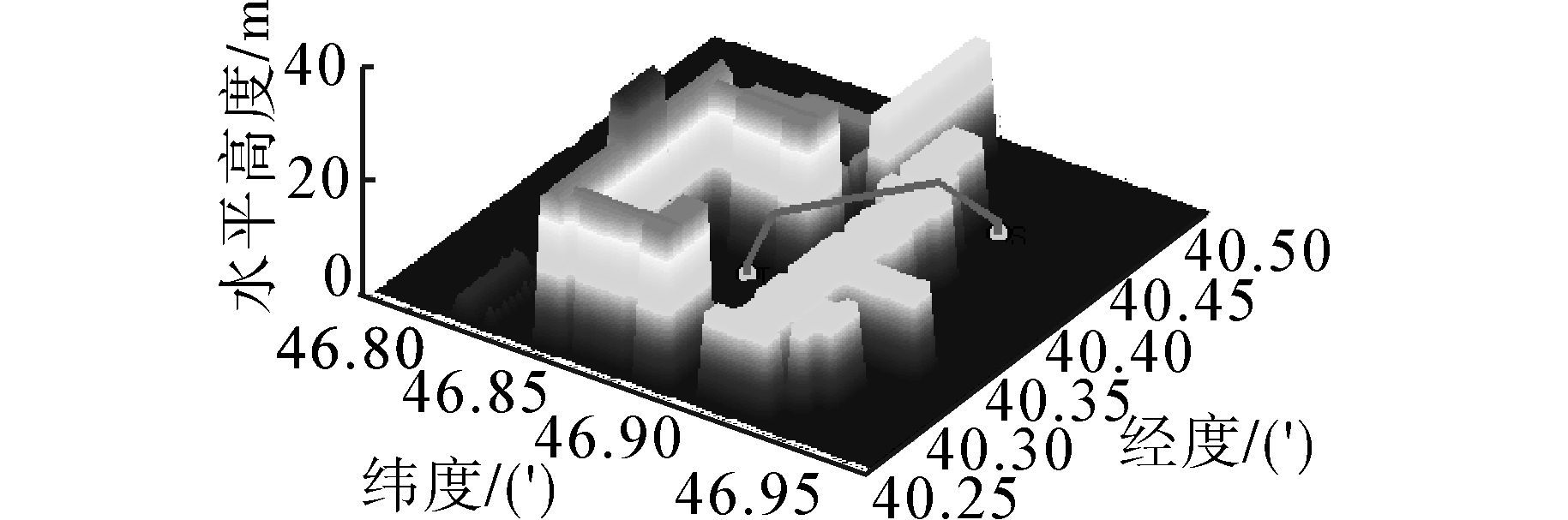图 22 蚁群算法搜索到的最优三维航迹图形Fig. 22 The best three-dimensional graphic searched by Ant colony algorithm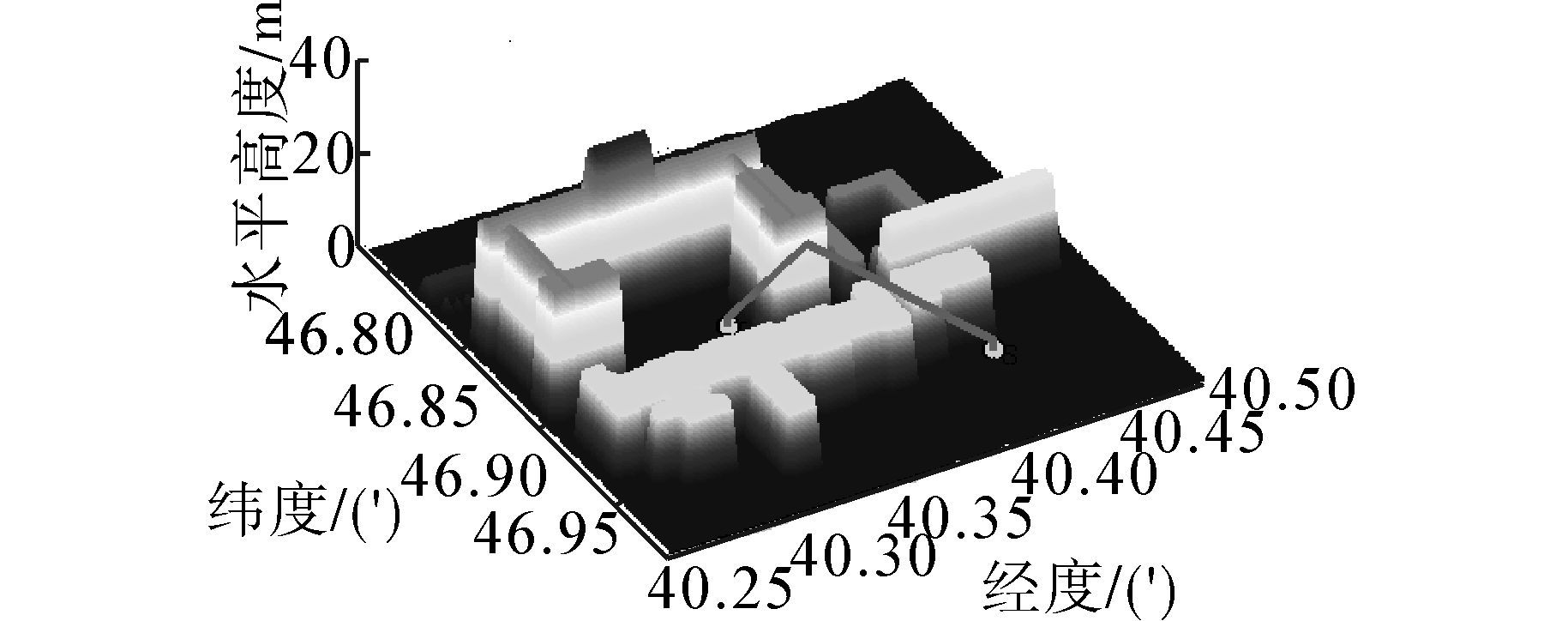图 23 粒子群算法搜索到的最优三维航迹图形Fig. 23 The best three-dimensional graphic searched by Particle swarm optimization

 $${S_{ae}} = ({S_{\max }} - {S_{min}})/2$$ (18)

 $$S = \sum\limits_{i = 1}^m {{S_i}/m}$$ (19)

 $$\sigma = \sqrt {{{\sum\limits_{i = 1}^m {{{({S_i} - {S_{mean}})}^2}} } \over m}}$$ (20)

 算法 最小值 最大值 均值 中值 标准差 ACO 225.958 238.094 232.224 232.658 2.665 PSO 218.996 284.540 248.474 239.351 22.283

 $$e = {{{S_{mean}} - S * } \over {S * }} \times 100\%$$ (21)

 算法 理论最优值 均值 误差率/% ACO 218.966 232.223 6.055 PSO 218.966 248.474 13.476

 算法 最小值 最大值 均值 中值 标准差 ACO 20.091 20.831 20.457 20.440 0.141 PSO 0.026 0.578 0.228 0.171 0.162

5 结束语

  METEA M, TSAI J. Route planning for intelligent autonomous land vehicles using hierarchical terrain representation[C]//Proceedings of IEEE International Conference on Robotics and Automation. Raleigh, NC, USA, 2010: 1947-1952.  谢奉军, 张丹平, 黄蕾,等. 旋翼无人机专利申请现状与技术发展趋势分析[C]//第六届中国航空学会青年科技论坛文集. 沈阳, 2014.  LIU Hongyun, JIANG Xiao, JU Hehua. Multi-goal path planning algorithm for mobile robots in grid space[C]//Proceedings of the 25th Chinese Control and Decision Conference. Guiyang, 2013: 2872-2876.  GALCERAN E, CARRERAS M. A survey on coverage path planning for robotics[J]. Robotics and Autonomous Systems, 2013, 61(12): 1258-1276.  李翊, 王朕, 姜鹏, 等. 四旋翼无人飞行器的航迹规划[J]. 舰船电子工程, 2014, 34(12): 58-61.LI Yi, WANG Zhen, JIANG Peng, et al. Path planning of quad-rotor[J]. Ship electronic engineering, 2013, 61(12): 1258-1276.  王玥, 张志强, 曹晓文. A*改进算法及其在航迹规划中的应用[J]. 信息系统工程, 2014 (1): 89-91.  韩超, 王赢. 一种基于改进PSO的无人机航路规划方法[J]. 舰船电子工程, 2014, 34(4): 49-53. HAN Chao, WANG Ying. Path planning of UAV based on an improved PSO algorithm[J]. Ship electronic engineering, 2014, 34(4): 49-53.  胡小兵, 黄席樾. 基于蚁群算法的三维空间机器人的路径规划[J]. 重庆大学学报, 2004, 27(8): 132-135.HU Xiaobing, HUANG Xiyue. Path planning in 3-D space for robot based on ant colony algorithm[J]. Journal of Chongqing university: natural science edition, 2004, 27(8): 132-135.  胡中华. 基于智能优化算法的无人机航迹规划若干关键技术研究[D]. 南京: 南京航空航天大学, 2011: 46-52.  KHATIB O. Real-time obstacle avoidance for manipulators and mobile robots[J]. International Journal of Robotics Research, 1986, 5(1): 90-99.  BAIRD C, ABRARNSON M. A comparison of several digital map-aided navigation technique[C]//The Proceedings of IEEE PLANS. Halifax, Nova Scotia, Canada, 1984: 286-293.  任世军, 洪炳熔, 黄德海. 一种基于栅格扩展的机器人路径规划方法[J]. 哈尔滨工业大学学报, 2001, 33(1): 68-72.REN Shijun,HONG Bingrong, HUANG Dehai. A robot path planning algorithm based on grid expansion[J]. Journal of Harbin institute of technology, 2001, 33(1): 68-72.  吕太之, 赵春霞. 基于改进概率栅格分解的路径规划算法[J]. 计算机工程, 2007, 33(21): 160-162, 165.LV Taizhi,ZHAO Chunxia. Path planning based on improved probabilistic cell decomposition[J]. Computer engineering, 2007, 33(21): 160-162, 165.  王凌. 智能优化算法及其应用[M]. 北京: 清华大学出版社, 2001.  WEISS M A. 数据结构与算法分析[M]. 冯舜玺, 译. 2版. 北京: 机械工业出版社, 2004.
DOI: 10.11992/tis.201509009

0

#### 文章信息

MO Hongwei, MA Jingwen

Four-rotor route planning based on the ant colony algorithm

CAAI Transactions on Intelligent Systems, 2016, 11(02): 216-225.
DOI: 10.11992/tis.201509009